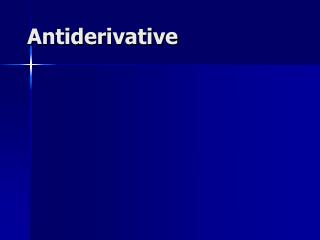DownloadDownload PresentationAntiderivative

Antiderivative

Download PresentationAntiderivative

- - - - - - - - - - - - - - - - - - - - - - - - - - - E N D - - - - - - - - - - - - - - - - - - - - - - - - - - -
Presentation Transcript

1. Antiderivative

2. Buttons on your calculator have a second button • Square root of 100 is 10 because • 10 square is 100 • Arcsin( ½ ) = p/6 because • Sin( p/6 ) = ½ • Recipicol of 20 is 0.05 because • Recipicol of 0.05 is 20

3. Definition: • F is an antiderivative of f on D if f is the derivative of F on D or F' = f on D.

4. Definition: • Name an antiderivative of f(x) = cos(x). • F(x) = sin(x) because the derivative of sin(x) is cos(x) or F’(x) = f(x).

5. G = F + 3 is also an antiderivative of f • because G' = (F + 3)' = f + 0 = f. • sin(x) and the sin(x) + 3 are both antiderivatives of the Cos(x) on R because when you differentiate either one you get cos(x).

6. G = F + k is also an antiderivative of f • How many antiderivatives of Cos(x) are there? • If you said infinite, you are correct. • G(x) = sin(x) + k is one for every real number k.

7. Which of the following is an antiderivative of y = cos(x)? • F(x) = 1 – sin(x) • F(x) = - sin(x) • F(x) = sin(x) + 73 • F(x) = cos(x)

8. Buttons on your calculator have a second button • A square root of 100 is -10 because • (-10) square is 100 • arcsin( ½ ) = 5p/6 because • Sin( 5p/6 ) = ½ • Recipicol of 20 is 0.05 because • Recipicol of 0.05 is 20

9. Theorem: • If F and G are antiderivatives of f on an interval I, then F(x) - G(x) = k where k is a real number.

10. Proof: • Let F and G be antiderivatives of f on I and define H(x) = F(x) - G(x) on I.

11. Proof: • Let F and G be antiderivatives of f on I and define H(x) = F(x) - G(x) on I. • H’(x) = F’(x) – G’(x) = f(x) – f(x) = 0 for every x in I. • What if H(d) is different than H(e)?

12. H(x) = F(x) - G(x) = k • If the conclusion of the theorem were false, there would be numbers d < e in I for which H(d) H(e).

13. H(x) = F(x) - G(x) = k • Since H is differentiable and continuous on [d, e], the Mean Value Theorem guarantees a c, between d and e, for which H'(c) = (H(e) - H(d))/(e - d) which can’t be 0.

14. H(x) = F(x) - G(x) = kH’(x) = 0 for all x in I H’(c) = (H(e) - H(d))/(e - d) This contradicts the fact that H’( c) must be zero. q.e.d.

15. Which of the following are antiderivatives of y = 4? • 4x • 4x + 2 • 4x - 7 • All of the above

16. Since antiderivatives differ by a constant on intervals, we will use the notation  f(x)dx to represent the family of all antiderivatives of f. When written this way, we call this family the indefinite integral of f.

17. Using our new notation, evaluate

18. Using our new notation, evaluate

19. = • True • False

20. Theorems because [x + c]’ = 1 What is the integral of dx? x grandma, x

21. . • c • 3x + c • x2 + c • x + c

22. Theorems If F is an antiderivative of f => F’=f and kF is an antiderivative of kf because [kF]’ = k F’ = k f and

23. Theorems

24. . • 12 x + c • 0 • - 24 x + c • 24 x + c

25. Theorem • Proof: • F(x) • F’(x) =

26. Examples

27. . • 3 x2 + c • 9 x2 + c • x3 + c • 3 x3 + c

28. Examples

29. . • 4/x + c • -4/x + c • 4/x3 + c • -4/x3 + c

30. Theorem If k(x) = 2cos(x) + 3x2 – 4, evaluate

31. Trigonometry Theorems

32. . • 2 sin(x) – 1/x + c • 2 sin(x) – 1/x3 + c • - 2 sin(x) – 1/x + c